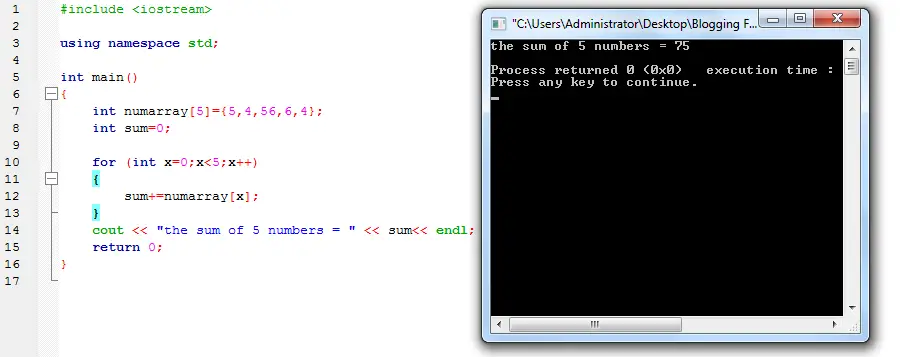# Sum of Numbers in an Array in C++• Version
• File Size 1.31 KB
• File Count 1
• Create Date May 9, 2016
• Last Updated May 9, 2016

# Sum of Numbers in an Array in C++

Sum of Numbers in an Array in C++

A sample program in c++ that will compute the sum of numbers in an array. The program will compute and display the sum of 5 numbers in an array. The program uses for loop to compute the sum and stops the loop when the condition is met.

Source code:

```#include < iostream >

using namespace std;

int main()
{
int numarray={5,4,56,6,4};
int sum=0;

for (int x=0;x<5;x++)
{
sum+=numarray[x];
}
cout << "the sum of 5 numbers = " << sum<< endl;
return 0;
}
```